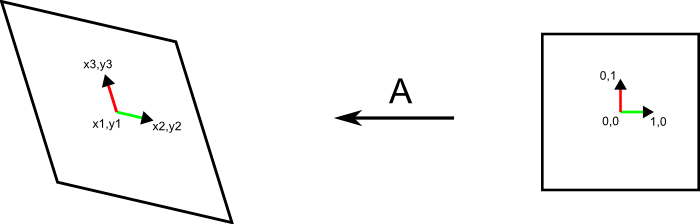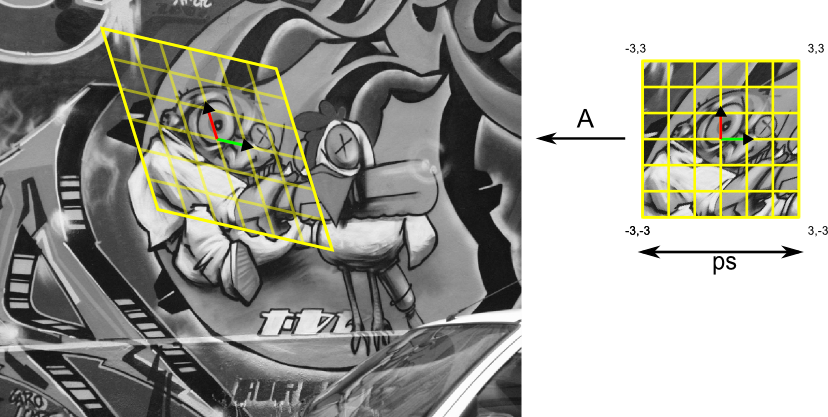Warning
This page is located in archive.

# Image Processing and Normalizing Transforms

## Convolution, Image Smoothing and Gradient

• The Gaussian function is often used in image processing as a low pass filter for noise reduction or so-called windowing function for weighting contribution of points in the neighbourhood. Write an implementation of the function G=gauss(x,sigma) that computes discrete samples of Gaussianin points given by vectorwith varianceand zero mean.
• Implement function D=dgauss(x,sigma) that returns a discrete samples of first derivative of Gaussianv in points given by vectorwith varianceand zero mean.
• Get acquainted with the function conv2.
• The effect of filtering with Gaussian can be studied on an impulse response:
sigma = 6.0;
x = [-ceil(3.0*sigma):ceil(3.0*sigma)];
G = gauss(x, sigma);
D = dgauss(x, sigma);
imp = zeros(51); imp(25,25) = 255;
out = conv2(G,G,imp);
...

imagesc(out); % or surf(out);
try to find out impulse responses of other combinations of the Gaussian and its derivatives.
• Write a function out=gaussfilter(in,sigma) that implements smoothing of an input image in with a Gaussian filter of width 2*(sigma*3.0)+1 and varianceand returns the smoothed image out (e.g. Lenna). Exploit the separability property of Gaussian filter and implement the smoothing as two convolutions with one dimensional Gaussian filter (see function conv2).
• Modify function gaussfilter to a new function [dx,dy]=gaussderiv(in,sigma) that returns the estimate of the gradient (gx, gy) in each point of the input image in (MxN matrix) after smoothing with Gaussian with variance. Use either first derivative of Gaussian or the convolution and symmetric difference to estimate the gradient.
• Implement function [dxx,dxy,dyy]=gaussderiv2(in,sigma) that returns all second derivatives of input image in (MxN matrix) after smoothing with Gaussian of variance.

## Geometric Transformations and Interpolation of the Image

• Implement function A=affine(x1,y1,x2,y2,x3,y3) that returns 3×3 transformation matrix A which transforms point in homogeneous coordinates from canonical coordinate system into image: (0,0,1)→(x1,y1,1), (1,0,1)→(x2,y2,1), (0,1,1)→(x3,y3,1).• Write function out=affinetr(in,A,ps,ext) that warps a patch from image in (MxN matrix) into canonical coordinate system. Affine transformation matrix A (3×3 elements) is a transformation matrix from the canonical coordinate system into image from previous task. The parameter ps defines the dimensions of the output image (the length of each side) and ext is a real number that defines the extent of the patch in coordinates of the canonical coordinate system. E.g. out=affinetr(in,A,41,3.0), returns the patch of size 41×41 pixels that corresponds to the rectangle (-3.0,-3.0)x(3.0,3.0) in the canonical coordinate system. Top left corner of the image has coordinates (0,0). Use bilinear interpolation for image warping. Check the functionality on image.## What should you submit?

Submit all the Matlab functions implemented in this lab: gauss.m, dgauss.m, gaussfilter.m, gaussderiv.m, gaussderiv2.m, affine.m and affinetr.m into the upload system in a .zip archive. Keep all functions in the root of the .zip archive together with all helper functions required. Follow closely the specification and order of the input/output arguments.

## Checking Your Results

You can check results of the functions required in this lab using the Matlab function publish. Copy the test script test.m into MATLAB path (directory with implemented functions) a run. Compare your results to the reference solution

courses/ucuws17/labs/02_improc/start.txt · Last modified: 2017/01/13 18:46 by prittjam